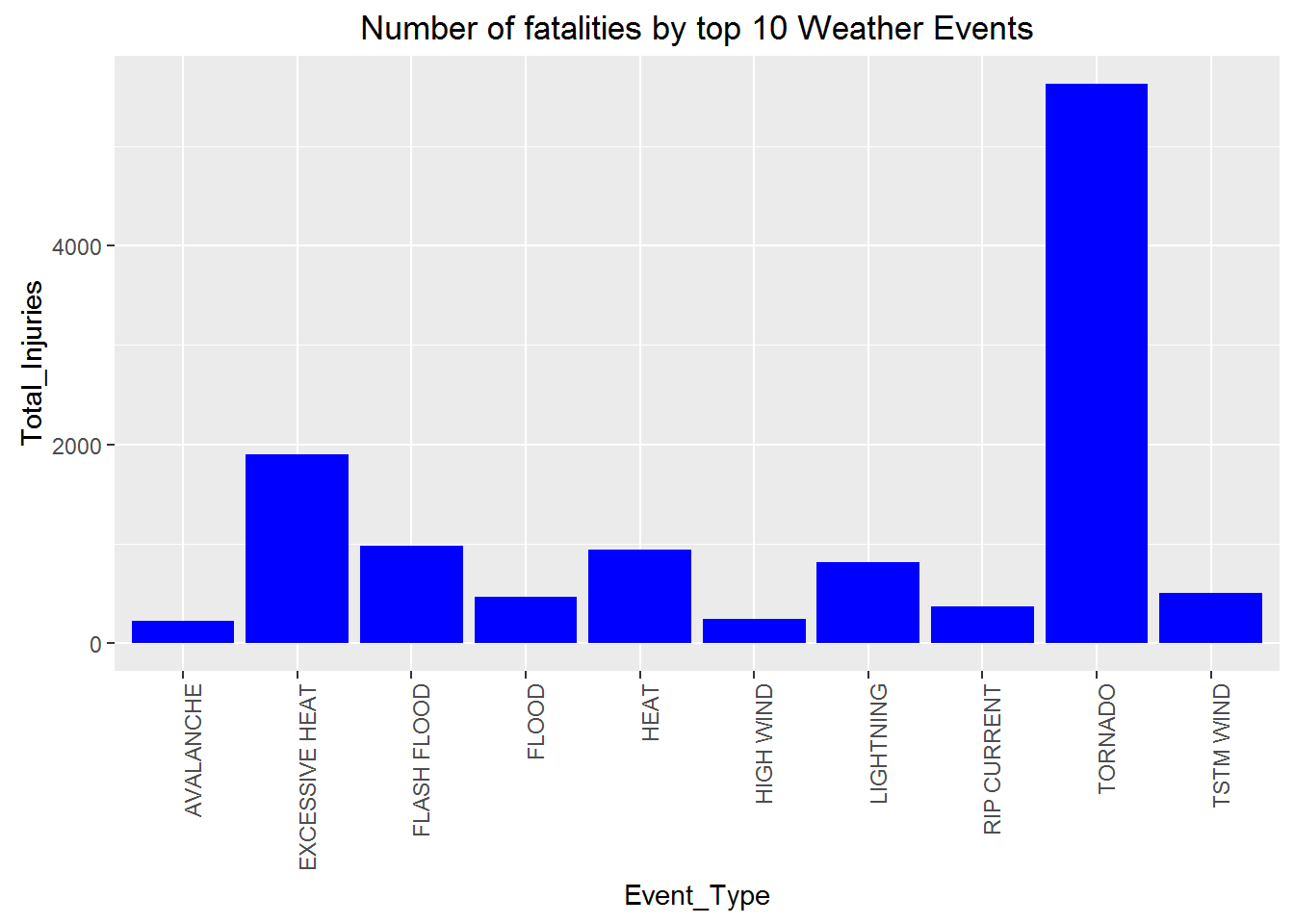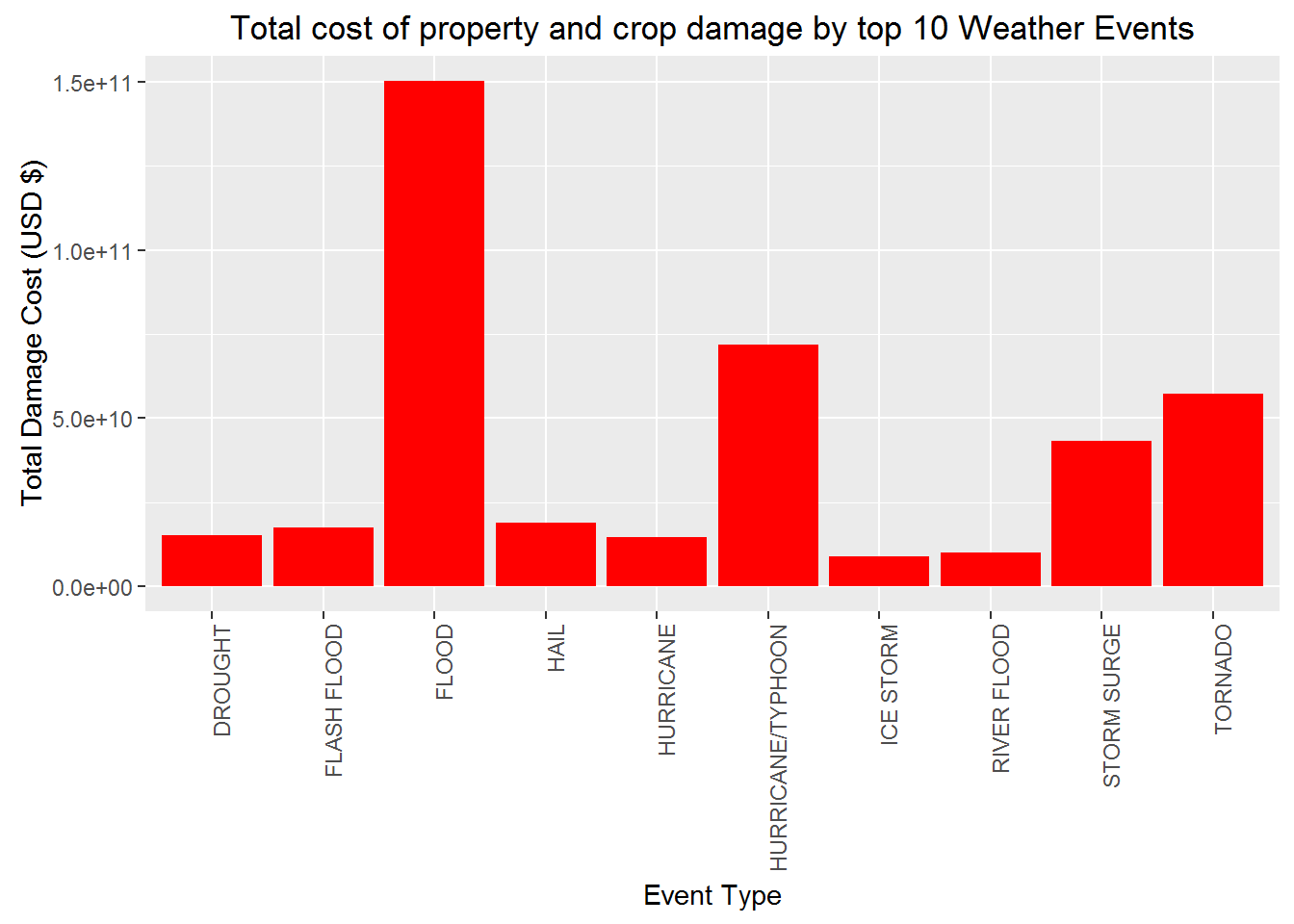## Synopsis:

1. Objective: explore the NOAA Storm Database to find out the most severe types of events(EVTYPE variable) with respect to population health and economic consequences.
2. Population health include two related variables: “FATALITIES(number of fatalities)”, “INJURIES(number of injuries)”.
3. Economic consequeces include four related variables: “PROPDMG(number of properties damaged)”, “PROPDMGEXP(exponent for property damage)”, “CROPDMG(number of crops damaged)”, “CROPDMGEXP(exponent for crop damage)”.
4. The report shows the top 10 weather event types for each index.

## Conclusion:

• Tornado is the most severe event type with respect to population health
• Flood is the most severe event type with respect to economic consequences.

## Data

• Dataset: U.S. National Oceanic and Atmospheric Administration’s (NOAA) storm database storm data
• This database tracks characteristics of major storms and weather events in the United States, including when and where they occur, as well as estimates of any fatalities, injuries, and property damage.
• The dataset is stored in a comma-separated-value file compressed via the bzip2 algorithm to reduce its size and there are a total of 902297 observations and 37 variables in this dataset

## Data Processing

###### environment setup
##      dplyr      tidyr    ggplot2      knitr   markdown downloader
##       TRUE       TRUE       TRUE       TRUE       TRUE       TRUE
##      sqldf
##       TRUE

#### summarise the top 10 fatal events types

df.event_fatalities_summary
##        Event_Type Total_Fatalities
## 2  EXCESSIVE HEAT             1903
## 3     FLASH FLOOD              978
## 4            HEAT              937
## 5       LIGHTNING              816
## 6       TSTM WIND              504
## 7           FLOOD              470
## 8     RIP CURRENT              368
## 9       HIGH WIND              248
## 10      AVALANCHE              224

#### Display the top 10 fatal events types

ggplot(df.event_fatalities_summary, aes(x = Event_Type, y = Total_Fatalities)) +
geom_bar(stat = "identity", fill = "blue", position = "stack") +
theme(axis.text.x = element_text(angle = 90, hjust = 1)) +
xlab("Event_Type") + ylab("Total_Injuries") + ggtitle("Number of fatalities by top 10 Weather Events")#### To calculate the property and crop costs - use the relative exponentor column to calculate the cost

unique.property.exp <- unique(df.indicators$PROPDMGEXP) unique.crop.exp <- unique(df.indicators$CROPDMGEXP)

df.indicators$PROPDMGCALC = 0 df.indicators[df.indicators$PROPDMGEXP == "H",]$PROPDMGCALC = df.indicators[df.indicators$PROPDMGEXP == "H",]$PROPDMG * 10 ^ 2 df.indicators[df.indicators$PROPDMGEXP == "K",]$PROPDMGCALC = df.indicators[df.indicators$PROPDMGEXP == "K",]$PROPDMG * 10 ^ 3 df.indicators[df.indicators$PROPDMGEXP == "M",]$PROPDMGCALC = df.indicators[df.indicators$PROPDMGEXP == "M",]$PROPDMG * 10 ^ 6 df.indicators[df.indicators$PROPDMGEXP == "B",]$PROPDMGCALC = df.indicators[df.indicators$PROPDMGEXP == "B",]$PROPDMG * 10 ^ 9 #### For crop damage introduce a new column called CROPDMGCALC - multiple the crop damage by its multiplier (H, K, M, B units) df.indicators$CROPDMGCALC = 0
df.indicators[df.indicators$CROPDMGEXP == "H",]$CROPDMGCALC = df.indicators[df.indicators$CROPDMGEXP == "H",]$CROPDMG * 10 ^ 2
df.indicators[df.indicators$CROPDMGEXP == "K",]$CROPDMGCALC = df.indicators[df.indicators$CROPDMGEXP == "K",]$CROPDMG * 10 ^ 3
df.indicators[df.indicators$CROPDMGEXP == "M",]$CROPDMGCALC = df.indicators[df.indicators$CROPDMGEXP == "M",]$CROPDMG * 10 ^ 6
df.indicators[df.indicators$CROPDMGEXP == "B",]$CROPDMGCALC = df.indicators[df.indicators$CROPDMGEXP == "B",]$CROPDMG * 10 ^ 9

#### Sum the property and crop total calculated cost into a new Total Damage Cost field and display the top 10

df.total_damage_costs <- sqldf("select EvType as Event_Type, sum(PROPDMGCALC) + sum(CROPDMGCALC) as Total_Damage_Cost from [df.indicators] group by Event_Type order by Total_Damage_Cost desc limit 10")

#### summarise the top 10 damage costs (shown in billions)

df.total_damage_costs
##           Event_Type Total_Damage_Cost
## 1              FLOOD      150319678250
## 2  HURRICANE/TYPHOON       71913712800
## 10         ICE STORM        8967041310
#### Display the top 10 property & crop events that caused the most damage in USD $ggplot(df.total_damage_costs, aes(x = Event_Type, y = Total_Damage_Cost)) + geom_bar(stat = "identity", fill = "red", position = "stack") + theme(axis.text.x = element_text(angle = 90, hjust = 1)) + xlab("Event Type") + ylab("Total Damage Cost (USD$)") + ggtitle("Total cost of property and crop damage by top 10 Weather Events")